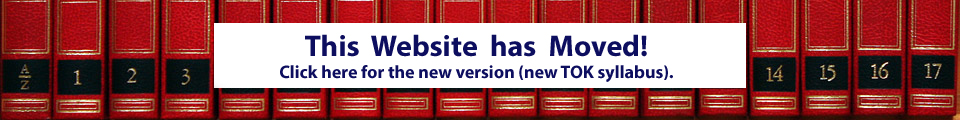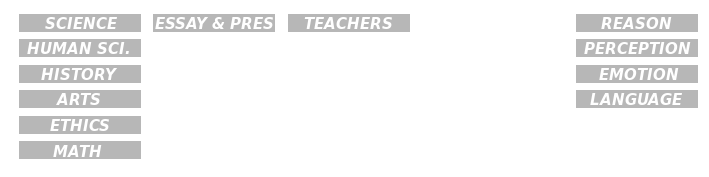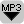## TOK Essay: Common ProblemsThere are some common, recurring problems with the TOK Essay. The prescribed title question is not answered explicitly, the examples are not explained, the essay is not organized, the….. Here is an overview of the most common problems.

The following audio files should give some basic advice for the TOK essay.

Some common TOK Essay problems are:

• Defining terms using only dictionary definitions without interpretation of the definition.
• Relevant ideas are not compared
• Use of generalizing statements and words: all, none, few, many….. It has always been known that…..
• Introductions that make not explicit reference to the topic, or introductions that do not properly interpret the topic question.

## What are Formal Systems?Introduced here: the MIU puzzle as an example of a formal system. A formal system is composed of axioms, to which rules of inference are applied to produce theorems to which the rules can be applied again. Confused? Try to MIU puzzle yourself – it’s fun!

The MU Puzzle is an example of a formal system. The objective of the MU Puzzle is to try to reach the string MU starting from MI, using only these four rules:

• Rule 1: xI ? xIU. If there is an I at the end of the string of letters, then you can add a U. For example if your string is MI then you can change it into MIU. You can only add a U if the last letter is an I.
• Rule 2: Mx ? Mxx. You can double any string that follows the M. So if your string is MIU then you can double the IU after the M. You will then get MIUIU. We have doubled the IU.

## What are the differences between Facts, Theories and Opinions?The terms facts, theories and opinions are often confused. The objective of this edition is to clarify these terms. Facts are observations or measurements from an experiment, theories are the explanation of these facts, while opinions are assessments or evaluations.

The terms facts, theories and opinions are often confused. The objective of this edition is to clarify these terms. Facts are observations or measurements from an experiment, theories are the explanation of these facts, while opinions are assessments or evaluations. Opinions are often not scientifically verifiable or falsifyable.

## What is Determinism?Determinism refers to the idea that the future is not up to chance, the future can be predicted. Determinists claim that if you had sufficient knowledge of the current situation, then you could in theory predict the behavior of a system into the future. Certain systems are indeed deterministic, many others are not.

In this edition we will have a look at the concept of determinism. In a deterministic system the initial conditions of the system determine the future behavior of the system. The behavior of both a falling apple and a rolling die depend on the initial conditions. Even the same laws of physics apply, namely classical mechanics. Both falling apple and the rolling dice are deterministic systems. But I am able to calculate the landing position of a falling apple, but I am not able to calculate the result of a die roll. Why? How are these two examples different? Why does the die seem to behave according to chance, but not a falling apple? A rolling die is chaotic and it is unpredictable, even though it is deterministic. Even if we know all the physical laws and the starting conditions of a system, it is still not possible to predict the future behavior of chaotic systems.